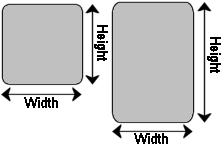PONTIAC POWER RULES ! ! !

# Calculator For Figuring Limiting Port Velocity Of Ports

Todays date is 9/25/2023

There exists a minimal port area for each combination that meets your target for peak rpm.
With almost every cylinder head (or intake) this power limiting port area is the constriction between the push rods
(or the most limiting intake runner cross section).
CA equals the minimum cross sectional area of your intake port. (height times width)
Port velocity is good, but too much will limit power - completely independent of CFM numbers

CA calculator at bottom of page.

CA:

Bore:

Stroke:

Peak RPM:

Input you Bore/Stroke and CA please

## To Figure Your Minimum Cross Sectional Area (CA) of your Rectangular Intake Port

 Make all measurements in inches, and convert fractions to decimal numbers. Examples: 1-1/4 = 1.25 2-3/16 = 2.1875 >> >> (14) + 1 (316) + 2

 Rectangular/Square runners To calculate the cross-sectional area of rectangular and square runner profiles, measure the width and the height of the runner, and multiply the two together.Measure in inches! Runner width: Runner height: Runner Area: square inches (calculated) Area = Width X Height

## To measure Round Ports

 Round runners To calculate the cross-sectional area of round runners, measure the diameter of the runner, divide by two, multiply the result by itself, then multiply that by 3.14.Measure in inches! Runner diameter: Runner Area: sq inches (calculated) Area = (Diameter2)2 X 3.14